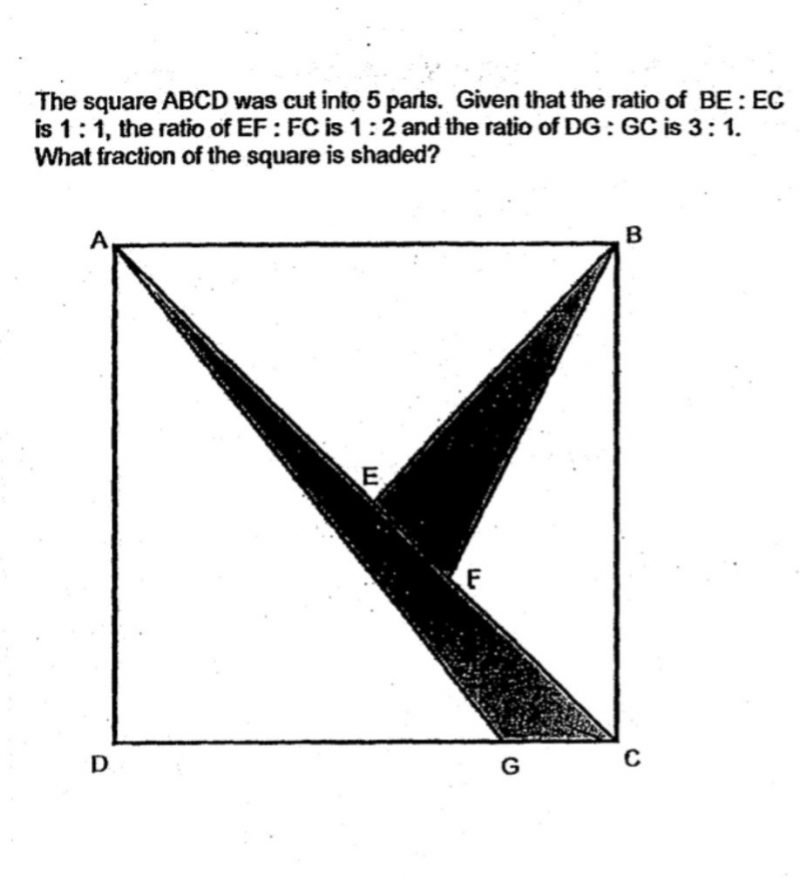Questionhi! can help us with this qn? we got 7/32 but answer sheet states answer as 5/24.

lets suppose ABCD=1, E must be the centre point of ABCD.

EF=EC/3, so BEF=BEC/3=1/12

0 Replies 0 Likes

5/24 is correct.
AGD = 3/4 * 1/2 = 9/24
ABF = 1/4 = 6/24
BFC = 2/3 * 1/4 = 4/24

Unshaded area = 9/24 + 6/24 + 4/24
= 19/24

Shaded area = 1 – 19/24 = 5/24

0 Replies 0 Likes